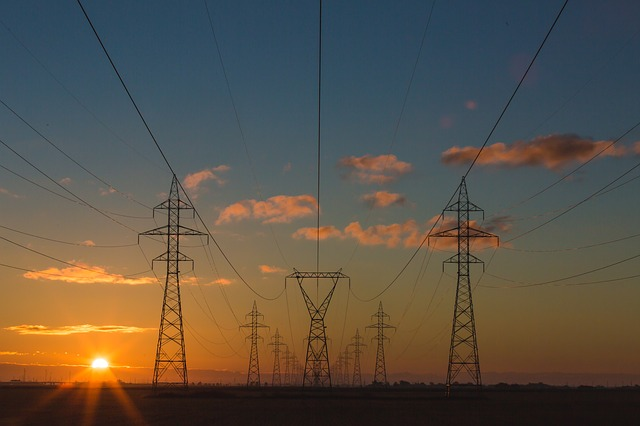µ GCSE | Charge

10 quick questions - for GCSE and iGCSE

10 minutes maximum! (can you do it in 5?)

1. Electrical charge is measured in

• A. amps
• B. volts
• C. ohms
• D. coulombs

2. The formula to calculate charge is

• A. charge = current x time ( Q = I.t )

• B. charge = current ÷ time    (Q = I/t )

• C. charge = current ÷ voltage    (Q = I/V )

• D. charge = voltage x current     ( Q = V.I )

3. When a charge flows along a wire in a circuit, the particles moving round are

• A. electrons
• B. protons
• C. neutrons
• D. atoms

What are the missing values in this table showing the charge flowing in a circuit?

 Charge Current Time 4. ? 0.2 C 2 C 5 C 100 C 20 A 5 s 5. 5 C 10 A ? 0.5 s 2 s 50 s 100 s 6. 400 C ? 0.1 A 1 A 16 A 1.6 MA 4000 s 7. ? 3.33 C 12 C 30 C 12000 C 200 mA 60 s

8. What charge flows through a power cable if 30 amps flows through it in 10 seconds?

• A. 3000 C
• B. 300 C
• C. 3 C
• D. 0.33 C9. A kettle needs a total charge of 1200 C to boil, in a time of 2 minutes. The current flowing in this time was

• A. 1 A
• B. 10 A
• C. 600 A
• D. 144 kA

10. This circuit was switched on for a short time and 0.4 C of charge flowed. How long was it on for?• A. 200 seconds
• B. 0.2 seconds
• C. 0.8 seconds
• D. 0.004 seconds